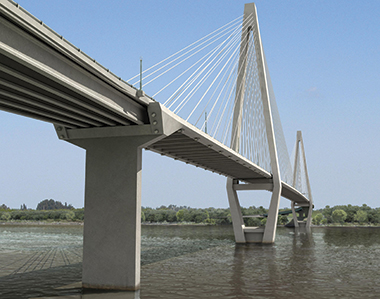Prestressed Concrete (PSC) structures especially bridges are being largely used all over the world due to their multifarious advantages in terms of structural behaviour, economy as well as aesthetical aspects. However, many PSC structures deteriorate with time due to several factors which may lead to certain loss in their initial applied prestress. This loss may affect the structure’s behaviour and its service life adversely and need to be checked. Such loss of prestress results in degradation of stiffness of the structure. A precise determination of prestress losses in prestressed concrete members is a complicated problem because the rate of loss due to one factor, such as relaxation of tendons, is continually being altered by changes in stress due to other factors, such as creep of concrete, fatigue, etc. It has been known that any change in the stiffness of the structure will change its natural frequency also. Hence, it may be possible to ascertain the loss by measurement of natural frequency at regular intervals. In this study, an effort has been made to know the natural frequency of a PSC structure by Vibration Method and determining the existing prestressing force to ascertain the loss of prestress.

Kumar Shashi Bhushan, Technical Officer, Dr. Rajeev Goel, Senior Principal Scientist, CSIR-CRRI, New Delhi, Dr. Awadhesh Kumar, Associate Professor, DTU, New Delhi.

Introduction

Prestressed Concrete (PSC) is defined as concrete in which there have been introduced internal stresses  of such magnitudes and distribution that the stresses resulting from given external loading are counteracted to a desired degree. Pre-stressed concrete (PSC) structures are advantageous in terms of delaying cracks, saving materials, reducing deflection etc.

However, there is substantial difference between the desired and the actual prestressing forces in existing PSC structures. Hence, this difference leads to severe and critical serviceability and safety problems. This difference in prestressing force is due to losses in prestressing force over a period of time. It is known that the loss of the prestress force in tendon occurs due to elastic shortening and bending of concrete, creep and shrinkage of concrete, steel relaxation, anchorage slip, and frictional loss between tendon and its surrounding materials. Also, the loss of the prestressing force unexpectedly occurs due to damage or breakage of prestressing strands. If this loss in the prestress is deviating from the estimated loss in the design, the structure will not behave in a manner as envisaged during the design. Therefore, it is very important to estimate the prestress-loss by considering the fact that a prestressed concrete member should keep effective force at each significant level of loading, together with appropriate material properties for that time during the service life of the structure.

To have trouble free service from pre-stressed concrete structures, lot of considerations are required at the time of construction. In the old existing bridges, durability aspect was usually ignored. Because of this, many times PSC structures develop defects  during their service life. The most common problems are corrosion of reinforcing steel and pre-stressing cables, cracks development, deflection, shrinkage of concrete, creep of concrete, etc. These defects may lead to loss of prestress force. It is important to note that there is no direct method to measure loss of pre-stress in old existing structures, where initial design data are not available.

Several researchers tried nondestructive evaluation methods such as vibration test  to estimate prestress loss in the PSC bridges. Based on the various researches, following is inferred :
• Loss of the prestress force in the structure results in the change in structural stiffness.
• Loss of the prestress force changes vibration characteristics of the structure.
• The change in structural stiffness can be estimated by monitoring changes in vibration characteristics of the structure.
Due to these, theoretical and experimental approach by using Rayleigh Method i.e. assuming conservative system of energy, correlating prestressing force and natural frequency is adopted for assessment in this study.

1. Cracking is highly reduced or eliminated.
2. Applying the prestressing forces deviating from the neutral axis induces moments which oppose those caused by externally applied loads, thus significantly reducing the deflection.
3. Smaller cross-section is required compared to RCC for the same imposed loads.
4. Smaller foundations due to reduction in self weight
1. The need for experienced & expert builders & engineers.
2. Initial cost of required equipment is very high.
3. Prestressed sections are brittle sections and are less fire resistant.
Prestress Losses:

Pre-stress loss is nothing but the reduction of initial applied prestress force in the cable with respect to time. In other words, loss in prestress is the difference between initial prestress and the effective prestress that remains in a member at a particular instant of time. Fig.ure-1 shows various losses occurred in the prestressing force immediately and over a period of time . The present study is concerned with losses occurred over a period of time in Post-tensioned systems of prestressing.

Also, the various types of losses in prestressed systems can be categorized as per the occurrence of tensioning of tendons carried out before or after casting of concrete i.e. Pre-tensioning or Post-tensioning systems of prestressing as tabulated in Table-1.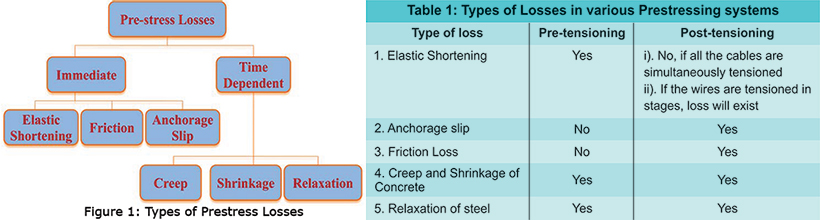In the present study, an attempt has been made to find the relation between natural frequency and prestressing force of a structure for determining the present prestressing force and consequently loss of prestress . Theoretical study is carried out to generalize a relation between prestressing force (P) and fundamental natural frequency (ω1) of the structure. The evaluation of prestressing force in terms of natural frequency is utilized because it is feasible to extract the natural frequency of an existing as well as new structure with the help of instrumentation and other techniques.

So, by analyzing a mathematical inter-relationship between first fundamental natural frequency (ω1) and prestressing force (P) for a particular type of structure, it is possible to estimate the existing force in PSC structure if its frequency is known. The present study is done in accordance with a study conducted by Wang et al  and by using Rayleigh Method. The theoretical approach used in the referred paper is based on Rayleigh’s method for getting an approximate value of natural frequency of a beam. Exact solutions of the model analysis problems are usually too cumbersome to obtain. In such situations, approximate methods can provide sufficiently accurate results to serve the purpose. Rayleigh’s method can be used to estimate the lowest (or fundamental) frequency of a self-adjoint (conservative) continuous system.

Details of Specimen:

Test model required for the study is available at Dynamic Heavy Testing laboratory of CSIR-CRRI at Ghaziabad. The bridge model was constructed in the year 2007. The superstructure for 10 m effective span bridge consists of two longitudinal PSC girders with 0.2m thick cast-in-situ RCC slab above the girders. The PSC girders are simply supported on end supports. The concrete grade adopted for the PSC girder is M45 and for the cast-in-situ RCC slab is M30 . The prestressing in each PSC girder is done using two cables consisting of 12T13. The cables are provided with 75 mm inner diameter (ID) and 81mm outer diameter (OD) of Corrugated HDPE sheathing. The correct cross section of PSC girder and plan showing locations of accelerometers on top of composite bridge model shown in Fig. 2&3. Firstly, an effort has been made to extract the present prestressing force in the cables of this bridge from the embedded load cells. Then, the natural frequency of the bridge has been evaluated using vibration based experimental study.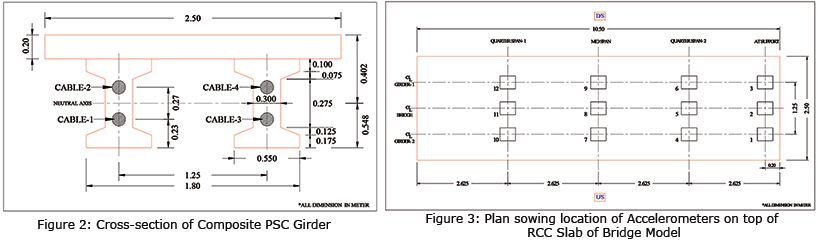Structural Detail:

Following are the detail of the test model employed for study:
• Effective span of girder (L) i.e. centre to centre of supports/bearings = 10.00 m
• Moment of Inertial of composite Section (I): 4810871.50 cm4
• Selected 7 ply 12.7 mm Φ (Low relaxation class-II strand, confirming IS 14268 : 1995)
• Each 12 T13 cable consists of 12 Nos. 12.7 mm Φ low relaxation strands
• The properties of prestress cables as given by the manufacturer are as follows:
• Area of each 12T13 cable: 1184.40 mm2
• Ultimate tensile strength: 1860.00 MPa
• Breaking Load of the cable: 2204.00 kN
• Anchorage slip at stressing end: 6mm
• As per IRC:112-2011, Modulus of elasticity of concrete (Ec ):
• Ec = Econcrete = 5000 √ fck MPa
• Ec (M-30) = 0.27386x105 MPa
• Ec (M-45) = 0.33541x105 MPa
• Ecj (j days) = 5000 √ fcj MPa
• Mass per unit length of the beam (ρ): 1.70 ton/m
• Es = 2.00 x 105 MPa
The cross sectional details of the composite girder showing tendon location are as shown in Fig.-2 and the plan showing location of sensors on the composite girder is shown in Fig.-3.

Experimental Study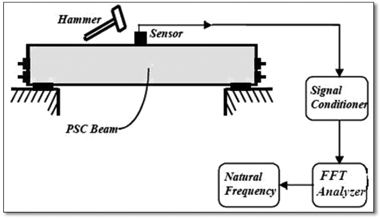Figure 4: Natural frequency measurement scheme
In this experimental study, the pre-stressing force present in all the four cables is measured using the Load Cells. The change in stress level can be known by periodical measurement of the same. The force in pre-stressing steel can be measured by using Vibrating Wire (VW) Load Cells or Vibrating Wire (VW) Strain Gauges. To achieve the objective of the study, experimental as well as theoretical studies have been carried out. In the experimental study, the prestressing force present in the cable is found out by deriving reading from the load cell, instrumented at the time of construction. This has been done by deploying read out unit (GK-403) for finding frequency and then calculating the load using equation. The natural frequency of first mode of beam is obtained using FFT analyzer after vibration study as shown in Fig.-4. While in the theoretical study, a relationship between first natural frequency of beam and prestressing force in cables of the beam has been established using Rayleigh‟s method. Finally a comparison between measured and calculated frequency is done for the feasibility of the adopted methodology.

Instrumentation of the Structure and Method adopted: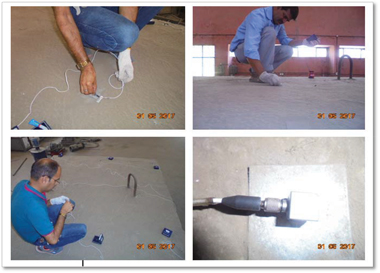Photo 1: Installation of accelerometers for vibration study
Twelve accelerometers (PCB Piezotronics model T333B50), OROS FFT Analyzer, and an impact hammer are used to know the natural frequency of the beam. The FFT or Fast Fourier Transform analyzer is one of a useful tool in vibrational analysis. The FFT analysis uses the Fast Fourier Transformation algorithm in which a continuous time domain gets converted to continuous frequency domain including magnitude as well as phase information. Accelerometer is an electromechanical device that measures the acceleration forces. These forces could be static, like the constant force of gravity, or they could be dynamic – due to moving or vibrating the accelerometer. For obtaining the natural frequency of the post-tensioned beam, the adopted procedure and set-up is shown in the Fig.-4, where the components of data acquisition system has been separately shown and the functions of each can be clearly understood.

The following steps have been used for the vibration study of the full scale composite bridge girder:
• The beam was cleaned and location for accelerometers marked.
• Grease is applied at the surface where sensors have to be placed in order to make perfect contact
• Twelve accelerometers were fixed as per the detail below (refer Fig.3.4):
• 3 numbers at Quarter span (towards roller end)
• 3 numbers at Mid span
• 3 numbers at Quarter span
• 3 numbers at support over rocker
• The connections were made between accelerometers, analyzer and displayer.
• Configuration setting has to be done for each and every accelerometer before installation of the same.
• The Girder was then subjected to hammering effect using the hammer.
• At supports, both quarters and mid span, hammering effect had been given on the top of the slab so that entire bridge may be covered.
• The Frequency vs Acceleration graphs were obtained using the displayer.
• The Frequency of the structure is then found out from the graph.
Theoretical Analysis of Composite Bridge girder:

Theoretical study is carried out to generalize a relation between prestressing force (P) and fundamental natural frequency (ω1) of the structure. So, by analyzing a mathematical inter-relation between first fundamental natural frequency (ω1) and prestressing force (P), it is possible to estimate the existing force in PSC structure if frequency is known . The theoretical approach used in the referred paper is based on Rayleigh’s method for getting an approximate value of natural frequency of a beam. Exact solutions of the modal analysis problem are usually too cumbersome to obtain . In such situations, approximate methods can provide sufficiently accurate results to serve the purpose. Rayleigh’s method can be used to estimate the lowest (or fundamental) frequency of a system. In this study, simply supported beam with parabolic tendon profile has been analyzed using the mathematical approach as shown in Fig.-5. The same mathematical approach is utilized to analyze the single span PSC composite girder, on which experimental studies have been carried out. This method is used to determine the approximate value of fundamental natural frequency. This method is based on the principle that if the system is conservative, the maximum kinetic energy (Tmax) is equal to the maximum potential energy (Vmax). By equating both Tmax and Vmax, the fundamental natural frequency ω1 can be obtained.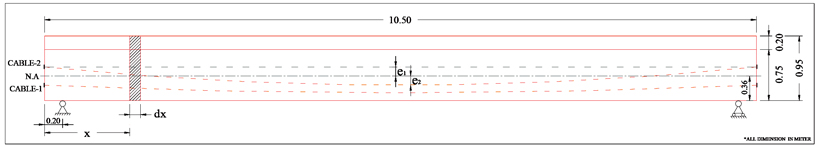Figure 5: Simply supported beam with parabolic duct profile

Natural frequencies of bridges have certain sensitivity to prestressing force , so the identification of effective prestressing force based on bridge’s natural frequencies is an effective method. The behaviour of prestressed beam can be described as a combination of two sub-systems: a compressive concrete beam and a tensioned cable. The dynamic equilibrium equations of the two sub systems are established using Rayleigh Method of structural vibration analysis. Based on the coupling analysis and processing of the vibration equation of sub systems, a calculation method of natural frequency of beam is derived and fundamental frequency can be calculated.

This method is used to determine the approximate value of fundamental natural frequency.

The kinetic energy of Prestressed Concrete Beam is expressed as: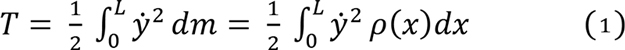Where, ρ(x) mass per unit length, y is the harmonic moving equation assumed as:
y = w(x) cosωt.
w(x) is assumed as vertical deformed shape function of the beam. Then the maximum kinetic energy (Tmax) can be expressed as: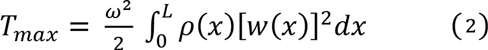By ignoring work done by shear forces, the potential energy of the deformed beam can be expressed as: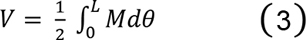Where M is the bending moment expressed as: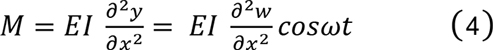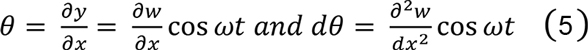By substituting M and dθ from equations (4) and (5) in (3), we get, the maximum value of V as: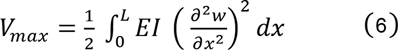By putting Tmax = Vmax, the fundamental natural frequency ‘ω1’ can be obtained as: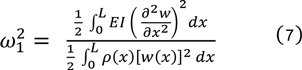In this case of considered specimen for vibration study, the approximate fundamental natural frequency can be obtained as: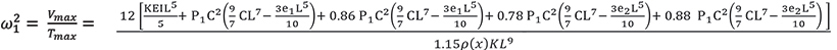Where,

ω1 = Fundamental Natural Frequency
E = Modulus of Elasticity
I = Moment of Inertial
K =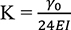where g0 is unit weight of the beam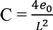P = Prestressing force
L = Effective Span of Girder
e1 & e2 = Eccentricity above and below neutral axis
ρ(x) = Weight of beam per running unit
By putting the values of EI, K, ρ, P, L, C1, C2, e1 & e2 in equation we get, ω12 = 35007.13
First Natural Frequency ‘f’ of structure = ω1 /2π = 29.93 Hz

Results

Measurement through read out unit has been carried out to find the values of the frequency from load cells and then converting it into prestressing force using the equation as per calibration certificate of load cells: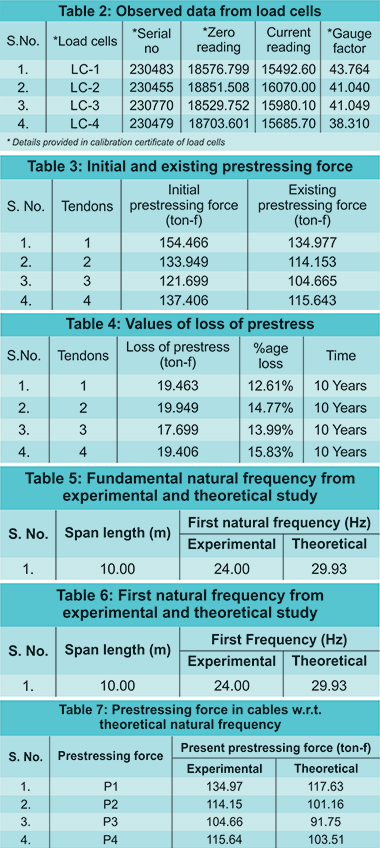The data obtained from load cells are tabulated in the Table-2 as below:

The results for existing and initial prestressing force in tendons are given in Table -3, loss of prestress along with percentage loss is calculated and tabulated in Table -4 as below.

Based on the initial applied prestressing force in tendons and the calculated existing prestressing force, loss of prestress can be worked out during last 10 years as given below in Table -4:

The loss observed is quite uniform throughout. The slight variation could be due to the application of successive prestressing in all the cables. So, there might be some loss of prestress occurred in the cables at the time of application of prestress. Spectrum of the vertical vibration (Frequency vs Acceleration) has also been processed out and some of the results have been shown in Graph-1 and 2. From the spectrum, results of estimation of first frequency of vibration for the span from this experimental study are found to be in the range of 24.00 Hz to 26.00 Hz. From the spectrum of the vertical vibration, it is observed that the natural frequencies for each location are different. It could be due to the reason of local stiffness because global excitation is probably can not achieved, so the effect of local stiffness of girders or low stiffness of the slab has made it of variable frequency. The theoretical and experimental values of fundamental frequency of structure are given in Table-5.

Results of estimation of first frequency of vibration for the span from this experimental and theoretical study are given in Table.6. From the graphs, it is found that the minimum frequency measured in vibration study is 24.00 Hz.

Theoretical and experimental results:

From the graphs, it is observed that the natural frequencies for each location are different. It could be due to the local stiffness because global excitation is probably could not achieved, so the effect of local stiffness of girders or low stiffness of the slab has made it variable frequency. From the theoretical value of natural frequency, the prestressing force P1 in the cables has determined and respective values for P2, P3 and P4 have calculated by using Table.3. The values for P1, P2, P3 and P4 are tabulated in Table.7.

Discussion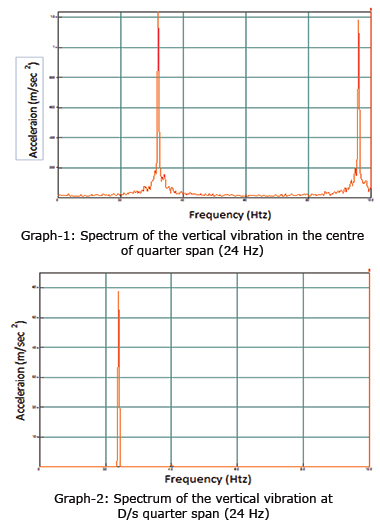Test results obtained from theoretical as well as experimental studies, it can be seen that the method used for estimating the natural frequency and prestressing force is quite satisfying and could be applicable for different PSC structures. However the result shows a variation of about 21% with respect to experimental results. This variation could be due to the following reasons:
• The material property of concrete changes with time, because of which certain parameters which depends on the material property of concrete like flexure rigidity will also get changed to some extent. Since these are being utilized in the analytical study, their variation could have affected the final result of estimated natural frequency.
• Fixity of the support bearings may get changed over a period of time due to various reasons, which in turn changes the bending moment of the beam. Since, in the analytical study, supports are assumed to be perfectly hinged and perfectly roller, so actual bending moments might be different than the bending moments computed assuming to be perfectly behaving supports.
• Spalling of the concrete has been observed in the beam at few locations. Thereby, the cross-section of the girders also gets altered from the designed girder section.
• In the theoretical analysis of beam, the value of modulus of elasticity is estimated on the basis of M30 and M45 grade of concrete (which was used in construction), disregarding the fact that after certain period of time the concrete gained some strength. The bridge has undergone fatigue testing of more than 1.4 million cycles due to which distress might have occurred.
Conclusions

The following conclusions have been derived from this study:
• Forces in all the prestressing strands are found to be less than the initial force applied to these strands about 10 years ago.
• The Fundamental frequency of single span prestressed concrete composite girder has been determined using vibration based damage detection technique and the fundamental frequency of structure obtained is in the range of 24.00 Hz to 30.00 Hz.
• Theoretical study has also been carried out to determine the natural frequency of the Structure and the frequency obtained as 31.63 Hz.
• The theoretically estimated natural frequency of the span is found to be more than the frequencies obtained from experimental study. This variation is between 15-20%, which could be due to change in boundary conditions, material properties, sectional properties, support condition, etc. over a period of time.
• With the passage of time, in future, the prestress will further reduce and correspondingly the fundamental frequency will also vary. Thus by keeping continuous or regular monitoring, the relation between prestressing force and fundamental frequency can be established which can be used as an effective non destructive technique of determining prestress level in a PSC structure.
Acknowledgement:

The authors are thankful to Director, CSIR-Central Road Research Institute, for providing the opportunity to carry out the work reported here.

References:
1. Halvonik, J., Dolnak, J., and Borzovic, V., “Long-term losses of prestress in Pre-cast members cast from HPC.” Concrete and Concrete structures conference, Elsevier, vol. 81-86, (2013).
2. Cawley, P., and Adams, R. D., “The location of defects in structures from measurements of natural frequency”, Journal of Strain Analysis, 80(8), 49-57 (1979)23. Clough, R.W., and Penzien, J., Dynamic of Structures, McGraw Hill. Instrumentation Techniques to Monitor Loss of Prestress and Corrosion of Steel in Pre-Stressed Concrete. Bridge and structure Report No.-34, RDSO, 21 June 2001.
3. Kim J. T., Ryu Y.S., and Yun C.B., “vibration-based method to detect prestress-loss in beam-type bridges.” Smart Structures and Materials, SPIE, vol. 5057 (2003).
4. Kim J.T., Na, W.B., Ryu, Y.S., Park, J.H., Lee, J.M., and Lee S.Y., “vibration based damage monitoring algorithms for prestress-loss in PSC girder Bridges” Sensors and Smart structure technologies for civil, mechanical and aerospace system, SPIE vol. 6932. (2008).
5. IS: 456:2000, Indian Standard Code of Practice for Plain and Reinforced Concrete, Bureau of Indian Standards, New Delhi, (2002).
6. Wang. T.H., Huang, R., and Wang, T.W., “The Variation of Flexural Rigidity for Post-Tensioned Prestressed Concrete Beams.” Journal of Marine Science and Technology, Vol. 21, No. 3, pp. 300-308 (2013).
7. Li, H., Lv, Z., and Liu, J., “assessment of prestress force in Bridges using structural dynamic responses under moving vehicles.” Mathematical problems in engineering, vol. 2013, (2013).
8. Jain, S.K., Murty, C.V.R., and Kamle, S., “Experimental Study on Natural Frequency of Prestressed Concrete Beams.” RDSO (2003).
9. Saiidi, M., Douglas, B., and Feng, S., “Prestress force effect on vibration frequency of concrete bridges”, Journal of Structural Engineering,, ASCE, 120(7), 2233-2241 (1994).
10. Yaoting, Z., and Ruige, L., “Natural Frequency of full pre-stressed concrete beam.” Transaction of Tianjan University, ISSN 1006-4982, vol. 13, No. 5, (October 2007).
To Know More or to Contact the Manufacturer
Invalid Input